Function Repository Resource:

# EfronBiasedCoin

Generate a restricted randomization between two groups, with a bias towards maintaining approximately equal allocation

Contributed by: Nicholas E. Brunk, Wolfram|Alpha Math Team
 ResourceFunction["EfronBiasedCoin"][p,n] generates a sequence of n restricted randomizations between two groups, maintaining approximately equal allocation with bias p.

## Examples

### Basic Examples (1)

Generate a sequence of 100 options with a bias of (p = 2/3) towards equal allocation:

 In:=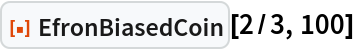Out=### Scope (3)

Different weighting can be given to the restriction of equal allocation:

 In:=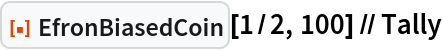Out=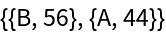In:=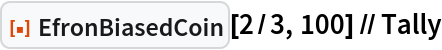Out=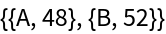In:=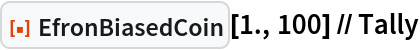Out=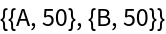Different sequence lengths can be generated:

 In:=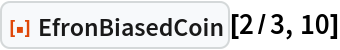Out=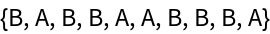In:=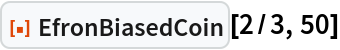Out=In:=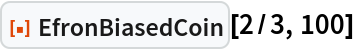Out=Users may replace the default symbols for the groups in any way they like:

 In:=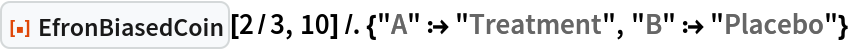Out=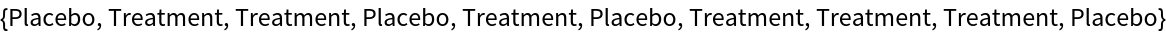### Properties and Relations (2)

Find the count of all values that follow an "A” when p is near 1/2:

 In:=Out=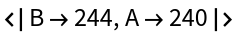Compare to when p is near 1. This shows a strong bias for alternating:

 In:=Out=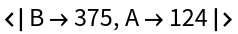### Neat Examples (4)

Complete randomization is achieved by a (p = 1/2) weighting towards selection of the underrepresented group:

 In:=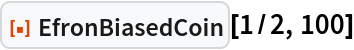Out=Complete randomization (p = 1/2) is thus equivalent to simple utilization of RandomChoice, albeit with the sequentially biased algorithm being slower:

 In:=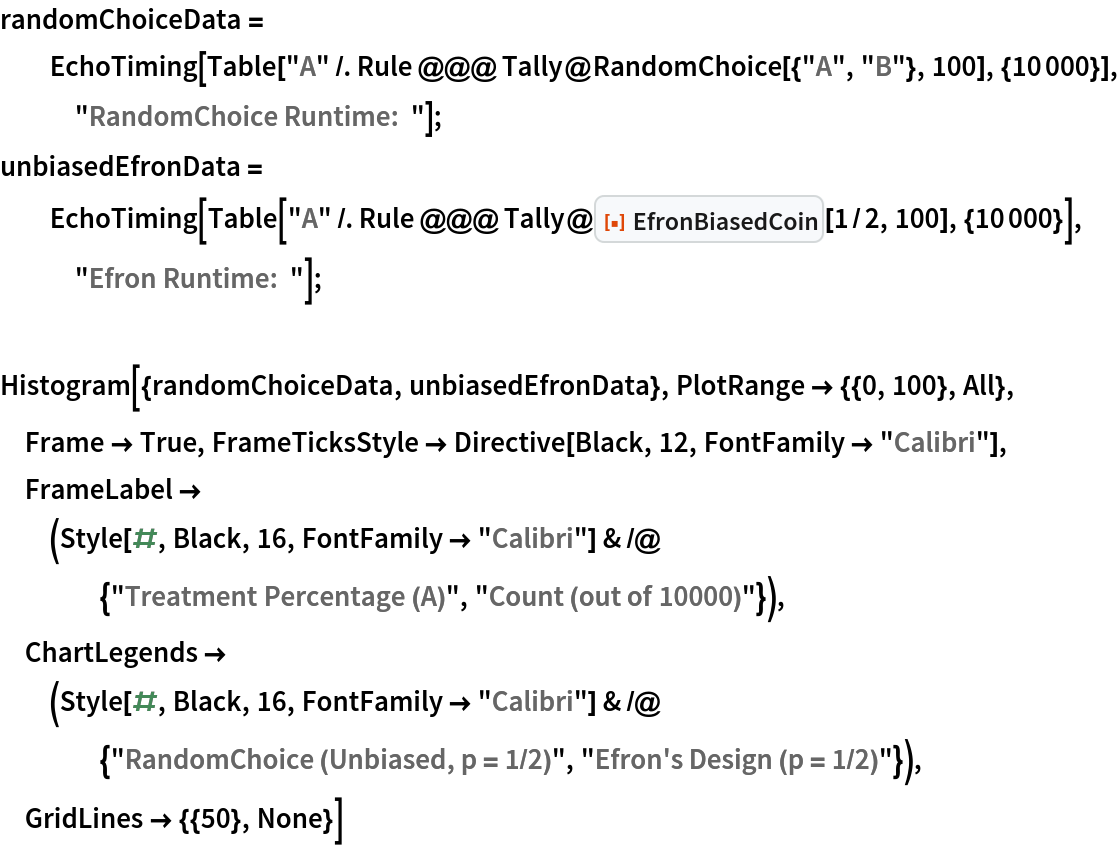"RandomChoice Runtime: " 0.1218419`"Efron Runtime: " 10.9277715`
 Out=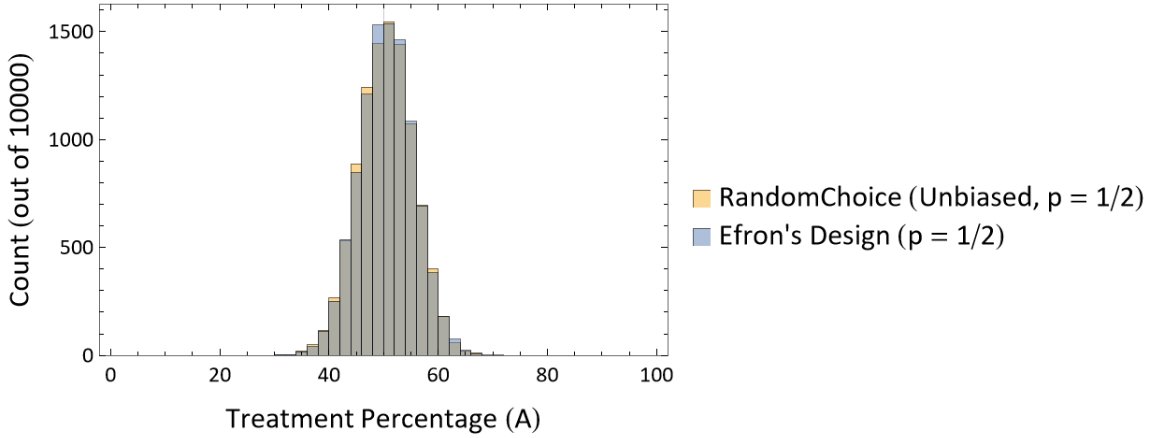In:=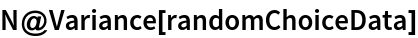Out=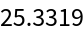In:=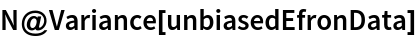Out=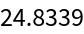The variance in allocation percentage for a given group can be diminished by weighting the randomization scheme (p ≠ 1/2):

 In:=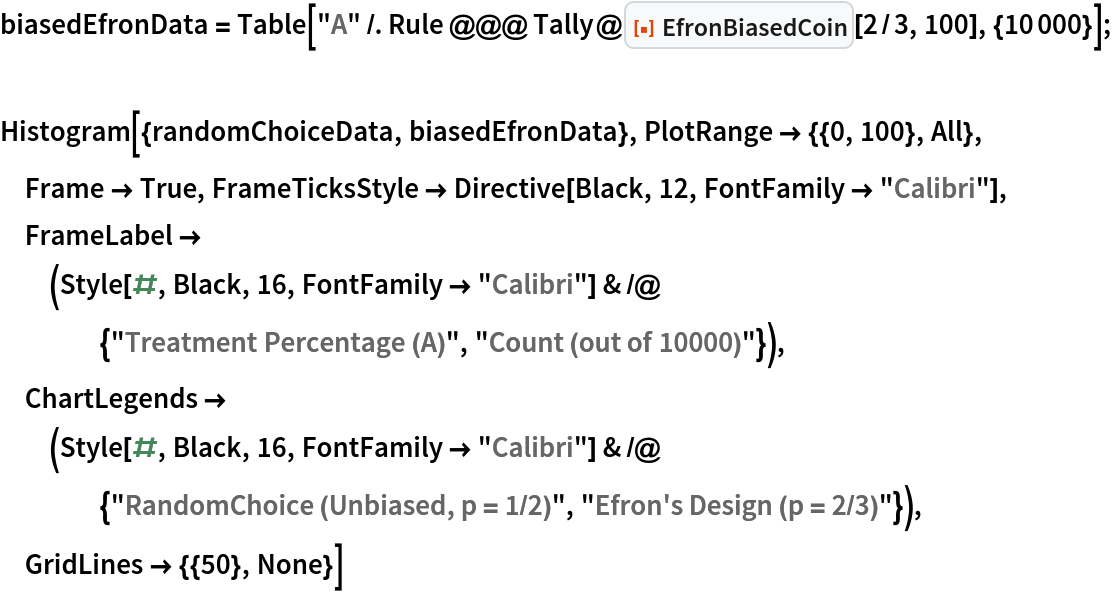Out=In:=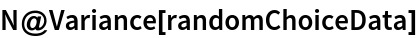Out=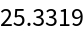In:=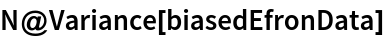Out=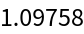Explore the options in variance of allocation percentage as a function of the bias p:

 In:=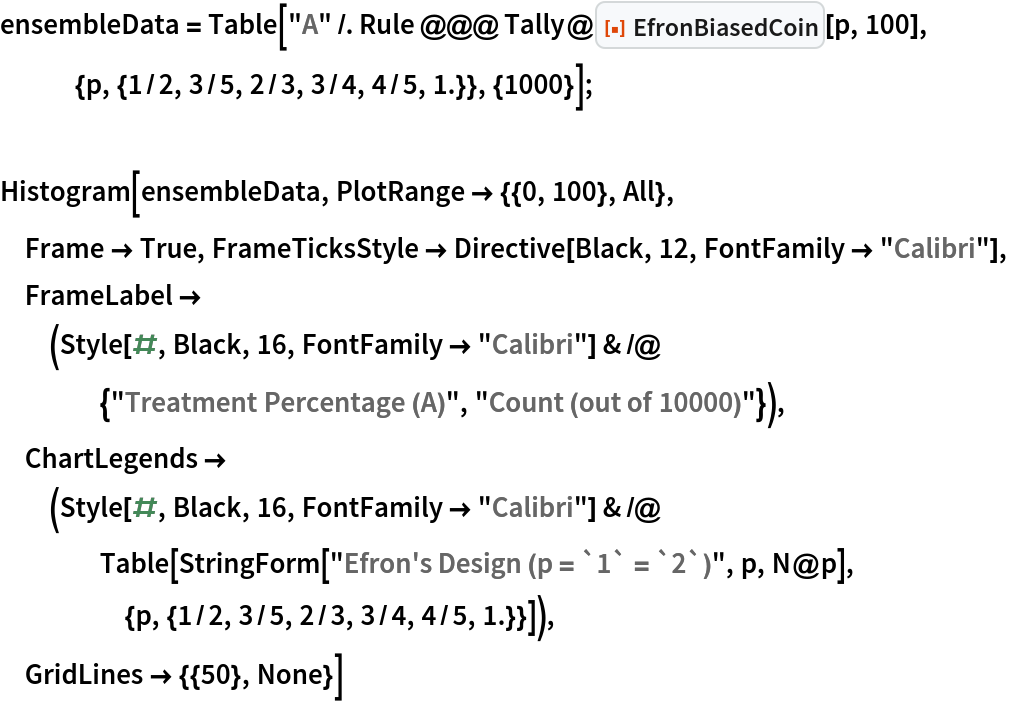Out=In:=Out=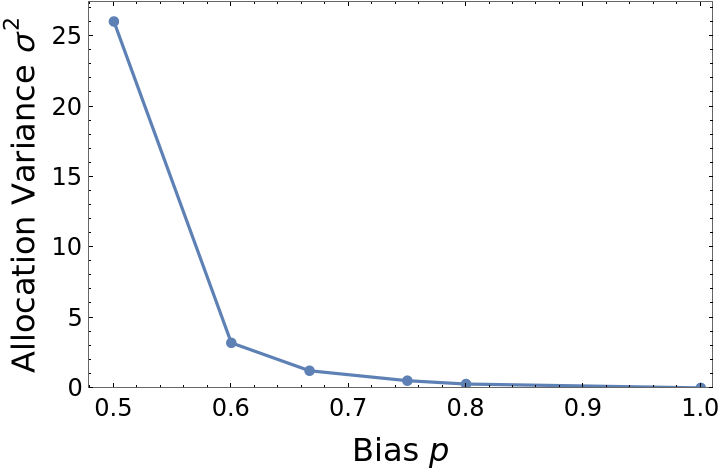## Publisher

Wolfram|Alpha Math Team

## Version History

• 2.0.0 – 23 March 2023
• 1.0.0 – 11 October 2022